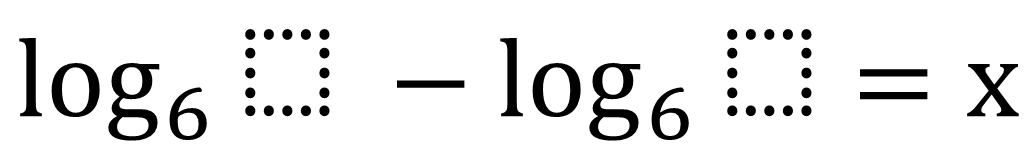# Properties of Logarithms

Directions: Using the integers 1 through 9, at most one time each, find the value of x that is closest to 0. Extension: Find more than one set of numbers that would make x = 0.### Hint

How can you rewrite this expression using properties of logarithms?

Source: Claire Verti

## Asymptotes of Rational Functions

Directions: Using the digits 1 to 9 at most one time each, place a digit …

1.Alexander Fischer

I love this question. Thank you for providing it! I would argue that log base six of eight ninths is the only correct answer however.

•Why do you think that log_6(8/9) is the only correct answer?

I’m having trouble seeing how that would be the case if log_6(8/9) and log_6(9/8) are opposites of one another.

2.1/9 is closer to 1 than 1/8. Do we need to revise this answer???

•True, 1/9 is closer to 1 than 1/8, but with the logs an interesting difference happens:
log(8/9)=log(8)-log(9)
log(9/8)=log(9)-log(8)